### Displacement and Strain: Simple Examples of Displacement Fields

### Rigid Body Displacement

A rigid body displacement is represented by a constant displacement vector at every point. The new (deformed) position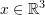of every point is related to the old (reference) position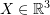as follows: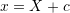where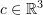is a constant vector. The displacement field at every point is the difference between the deformed and reference positions and is constant: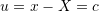Change the components of the vectorin the following tool to view its effect on the displacement of the cuboid.

### Rigid Body Rotation

A rigid body rotation is represented by a rotation matrix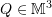(see Orthogonal Tensors) such that the new (deformed) positionof every point is equal to the rotation matrix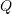multiplied by the old (reference) positionas follows: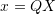The displacement field at every point is the difference between the deformed and reference positions: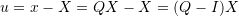Recall that any rotation matrix can be viewed as consecutive rotations around each of the basis vectors of the coordinate system. Clockwise rotations with anglesaround the basis vectors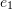,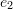and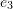are given by the following matrices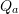,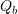and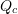, respectively: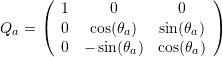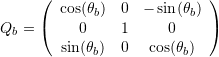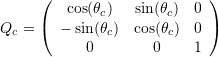It is important to notice that the order of rotation changes the final position of the rotated object. The rotation matrix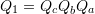describes a rotation of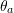aroundfollowed by a rotation of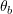aroundand finally a rotation of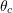around. On the other hand, the rotation matrix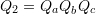describes a rotation ofaroundfollowed by a rotation ofaroundand finally a rotation ofaround. In general: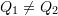. In the following example, the red box represents the original box after rotation around the basis vectors. Try it out: rotate the box 90 degrees aroundand then slowly rotate it around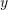. This order is applied to the image on the left. The order of rotation applied to the one on the right is reversed! Compare the two orders of rotation. The overall matrix of transformation is displayed at the bottom of each image.

### Rigid Body Motion

A rigid body motion is a combination of both a rigid body displacement and a rigid body rotation such that the deformed positionis function of the reference positionas follows: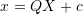whereis a rotation matrix andis a vector representing the rigid body displacement. The displacement field can be expressed as: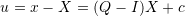In component form, the relationship between the vectorsand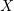can be written as follows: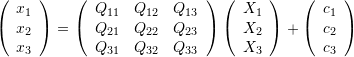Note that in some numerical analysis software and tools, the above relationship adopts the following form: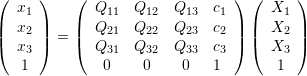Change the angles of rotation and the components of the vectorin the following tool to see the effect on the final position of the cube.

### Uniform Extension and Contraction

A uniform extension or contraction can be characterized by three positive parameters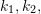and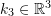that represent the ratios between the three vector components in the deformed configuration to the components in the reference configuration: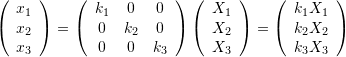Note that the relationship can be written as a linear transformation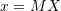where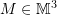has the form: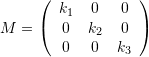In the following example, you can vary the values of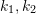and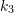to see the effect on the deformation of a cube. What values constitute compression and what values constitute tension? Also, what does it mean that the value of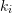is equal to 1 or 0?

### Simple Shear

The simple shear motion is described by a shearing angle along a certain direction and perpendicular to another direction. The following relationship describes a simple shear motion in which the planes parallel to the basis vectorsandare sheared in the direction of: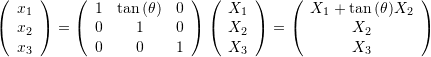Note that the relationship can be written as a linear transformationwherehas the form: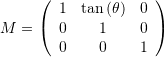In the following example, change the values of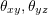and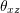in the matrix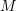: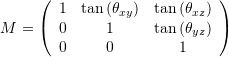and observe the effect on the deformation. The term simple shear applies to the deformations when only one of the anglesandis non-zero.

### Pure Shear

The following relationship describes a pure shear motion with an angle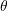in the plane ofand: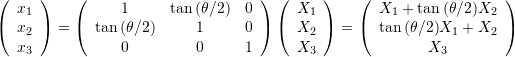Note that the relationship can be written as a linear transformationwherehas the form: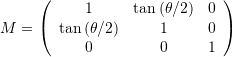The difference between pure shear and simple shear can be viewed in the following two dimensional example. Change the value of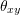to see the deformation of a rectangle under pure shear (on the left) and under simple shear (on the right). The matrixin each case is given underneath the figure:

In the following example, change the values ofandin the matrix: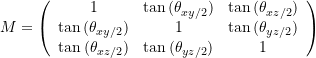and observe the effect on the deformation. The term pure shear applies to the deformations when only one of the anglesandis non-zero.